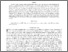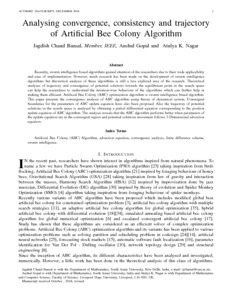# Analysing convergence, consistency and trajectory of Artificial Bee Colony Algorithm

Bansal, Jagdish Chand and Gopal, Anshul and Nagar, Atulya K. (2018) Analysing convergence, consistency and trajectory of Artificial Bee Colony Algorithm. IEEE Access. ISSN 2169-3536Preview
Text
Convergence of ABC.pdf - Accepted Version

Recently, swarm intelligence based algorithms gained attention of the researchers due to their wide applicability and ease of implementation. However, much research has been made on the development of swarm intelligence algorithms but theoretical analysis of these algorithms is still a less explored area of the research. Theoretical analyses of trajectory and convergence of potential solutions towards the equilibrium point in the search space can help the researchers to understand the iteration-wise behaviour of the algorithms which can further help in making them efficient. Artificial Bee Colony (ABC) optimization algorithm is swarm intelligence based algorithm. This paper presents the convergence analysis of ABC algorithm by using results from the theory of dynamical system and convergent boundaries for the parameters $\phi$ and $\psi$ is proposed. Also the trajectory of potential solutions in the search space is analysed by obtaining a partial differential equation corresponding to the position update equation of ABC algorithm. The analysis reveals that the ABC algorithm performs better/efficiently when parameters $\phi$ and $\psi$ are in the convergent region and potential solutions movement follows 1-Dimensional advection equation.View Item